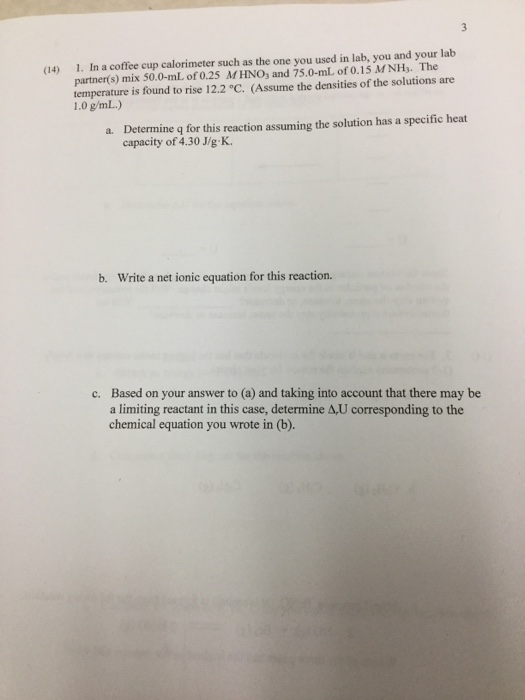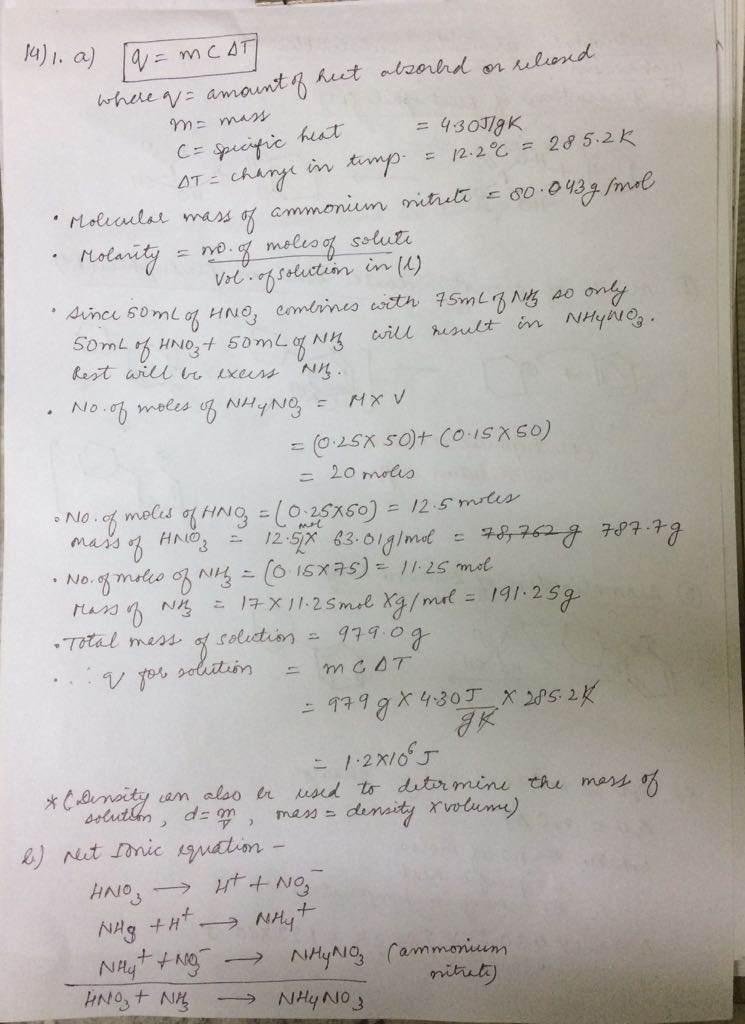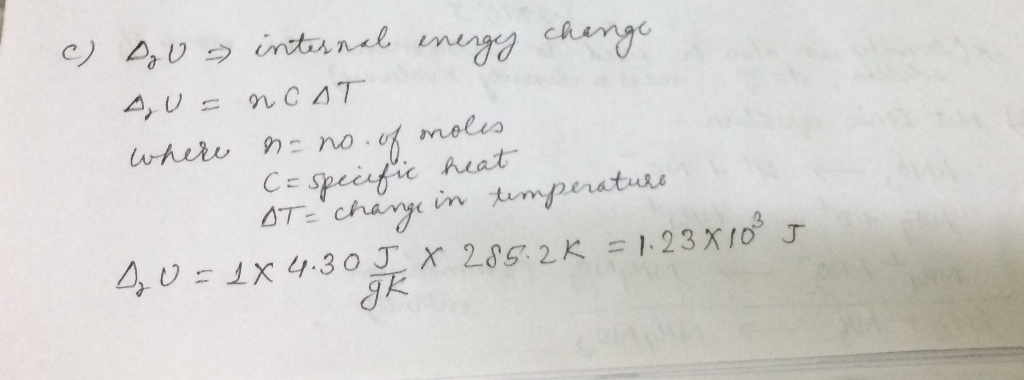# Question & Answer: In a coffee cup calorimeter such as the one you used in lab, you and your lab partner(s) mix…..In a coffee cup calorimeter such as the one you used in lab, you and your lab partner(s) mix 50.0-mL of 0.25 M HNO_3, and 75.0-mL of 0.15 MNH_3. The temperature is found to rise 12.2 degree C. (Assume the densities of the solutions are 1.0 g/mL.) a. Determine q for this reaction assuming the solution has a specific heat capacity of 4.30 J/g K. b. Write a net ionic equation for this reaction. c. Based on your answer to (a) and taking into account that there may be a limiting reactant in this case, determine delta _r U corresponding to the chemical equation you wrote in (b).